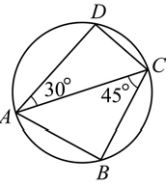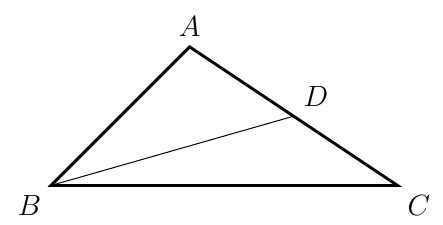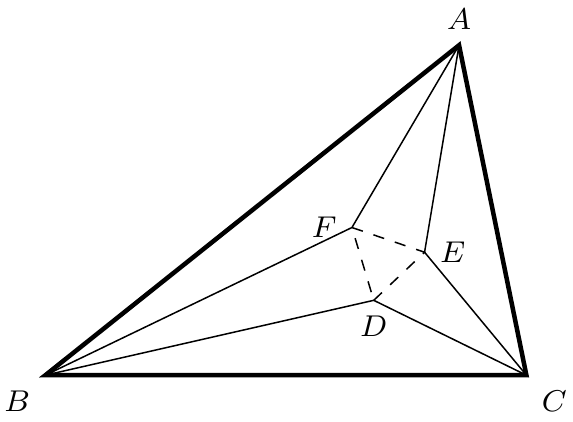###### back to index | new

In $\triangle{ABC}$, if $(b+c):(c+a):(a+b)=5:6:7$, compute $\sin{A}:\sin{B}:\sin{C}$.

Let quadrilateral $ABCD$ inscribe in a circle. If $\angle{CAD}=30^\circ$, $\angle{ACB}=45^\circ$, and $CD=2$. Find the length of $AB$.In $\triangle{ABC}$, if $\sin{A}:\sin{B}:\sin{C}=4:5:7$, compute $\cos{C}$ and $\sin{C}$.

Two people located 500 yards apart have spotted a hot air balloon. The angle of elevation from one person to the balloon is $67^\circ$. From the second person to the balloon the angle of elevation is $46^\circ$. How high is the balloon when it is spotted? (You can use a calculator. Please keep the result to the 2 decimal places.)

In $\triangle{ABC}$, if $(a^2 +b^2)\sin(A-B)=(a^2-b^2)\sin(A+B)$, determine the shape of $\triangle{ABC}$.

Let $\triangle{ABC}$ be an acute triangle and $a, b, c$ be the three sides opposite to $\angle{A}, \angle{B}, \angle{C}$ respectively. If vectors $m=(a+c,b)$ and $n=(a-c, b-a)$ satisfy $m\cdot n = 0$, (1) Compute the measurement of $\angle{C}$. (2) Find the range of $\sin{A} + \sin{B}$.

In $\triangle{ABC}$, if $a\cos{C} + \frac{c}{2} = b$, (1) compute $\angle{A}$. (2) if $a=1$, find the range of the perimeter o f $\triangle{ABC}$.

Let $BD$ be a median in $\triangle{ABC}$. If $AB=\frac{4\sqrt{6}}{3}$, $\cos{B}=\dfrac{\sqrt{6}}{6}$, and $BD=\sqrt{5}$, find the length of $BC$ and the value of $\sin{A}$.Let $R=\frac{7\sqrt{3}}{3}$ be the circumradius of $\triangle{ABC}$. If $\angle{B} = 60^\circ$ and its area $S_{\triangle{ABC}}=10\sqrt{3}$, find the lengths of $a$, $b$, and $c$.

In $\triangle{ABC}$, $AE$ and $AF$ trisects $\angle{A}$, $BF$ and $BD$ trisects $\angle{B}$, $CD$ and $CE$ trisects $\angle{C}$. Show that $\triangle{DEF}$ is equilateral.Let $O$ and $H$ be the circumcenter and orthocenter of $\triangle{ABC}$ respectively. Show that $OH\parallel BC$ if and only if $\tan{B}\tan{C}=3$.